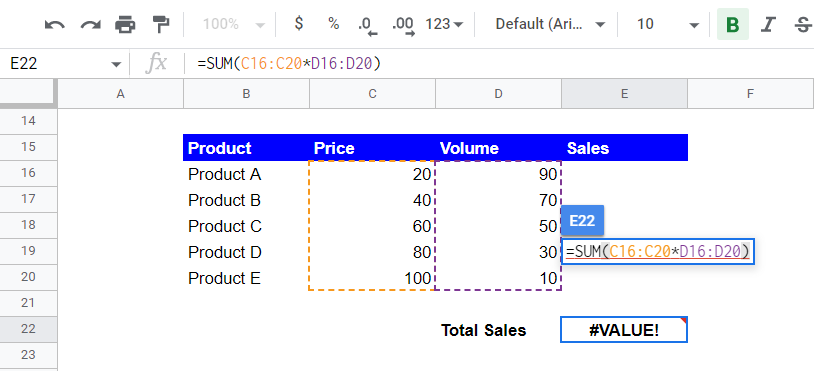# ARRAYFORMULA Function in Google Sheets: Explained

In this article, you will learn what the ARRAYFORMULA function is and how to use it.

## What is the ARRAYFORMULA function in Google Sheets?

Google’s explanation about the function of the ARRAYFORMULA is as follows: the ARRAYFORMULA Enables the display of values returned from an array formula into multiple rows and/or columns and the use of non-array functions with arrays.

What does this definition mean? The ARRAYFORMULA has the following two features:

1. The ARRAYFORMULA returns values across cells rather than a single value.
2. The ARRAYFORMULA allows you to input arrays in formulas that generally don’t accept arrays as an argument or don’t work correctly with arrays (non-array functions).

See examples in the later section and learn how it works more specifically.

## How to insert the ARRAYFORMULA function in Google Sheets

Standard way

1. Type “=ARRAYFORMULA” or navigate to the “Insert” tab (or “Functions” icon) → “Function”“Google”“ARRAYFORMULA”.
2. Insert a formula you want to run in the ARRAYFORMULA
3. Press the “Enter” key.

Faster way with a shortcut

1. Insert a formula(s) you want to run
2. Press “Ctrl”+”Shift”+”Enter” (for Windows) or “⌘”+”Shift”+”Enter” (for Mac), by which the ARRAYFORMULA encloses the inserted formula(s).
3. Press the “Enter” key.

The general syntax is as follows:

``=ARRAYFORMULA(array_formula)``

Array_formula: A range, mathematical expression using a cell or multiple ranges of the same size, or a function that returns a result larger than one cell. If you insert ranges, they must be the same size

## How to use the ARRAYFORMULA in Google Sheets

The ARRAYFORMULA returns values across cells rather than a single value.

Imagine you have a data set, as shown in the picture below. You want to calculate the sales amount of each product and the total sales.

As you want to reduce the number of copies and pastes, instead of conducting multiplication for the first row and copying and pasting it for the other products, you enter the formula, as shown in the screenshot below. You expect the formula to calculate each sales amount automatically. However, it doesn’t work as you wish because the basic operators, such as “+” and “*”, do not accept ranges as input.

You can utilize the ARRAYFORMULA here - Press “Ctrl”+”Shift”+”Enter” (for Windows), or “⌘”+”Shift”+”Enter” (for Mac) and push the “Enter” button. Now you have the ARRAYFORMULA, which automatically spreads its results into the other cells.

The ARRAYFORMULA allows non-array formulas to be used with arrays.

This time, you think it is excellent to get the total result from a formula expression in a cell. So, you enter the formula “=SUM(C16:C20*D16:D20)”, as shown in the following screenshot. However, it doesn’t act as expected because the SUM formula can’t accept multiple arrays as a single argument.An example of the SUM function that doesn’t work with arrays

Combining the ARRAYFORMULA and SUM formulas helps you get the result directly. With the SUM function in a cell, Press “Ctrl”+”Shift”+”Enter” (for Windows) or “⌘”+”Shift”+”Enter” (for Mac) and push the “Enter” button.